Next: General principle based priors Up: Choice of priors - Previous: Purely subjective assessment of

## Conjugate priors

Because of computational problems, modelling priors has been traditionally a compromise between a realistic assessment of beliefs and choosing a mathematical function that simplifies the analytic calculations. A well-known strategy is to choose a prior with a suitable form so the posterior belongs to the same functional family as the prior. The choice of the family depends on the likelihood. A prior and posterior chosen in this way are said to be conjugate. For instance, given a Gaussian likelihood and choosing a Gaussian prior, the posterior is still Gaussian, as we have seen in Eqs. (25), (28) and (29). This is because expressions of the form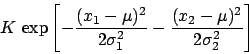can always be written in the form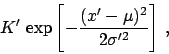with suitable values for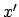,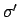and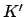. The Gaussian distribution is auto-conjugate. The mathematics is simplified but, unfortunately, only one shape is possible.

An interesting case, both for flexibility and practical interest is offered by the binomial likelihood (see Sect. 5.3). Apart from the binomial coefficient,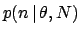has the shape, which has the same structure as the Beta distribution, well known to statisticians: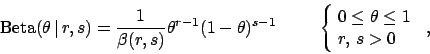(94)

where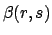stands for the Beta function, defined as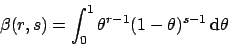(95)

which can be expressed in terms of Euler's Gamma function as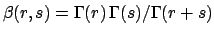. Expectation and variance of the Beta distribution are: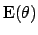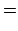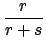(96)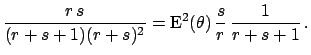(97)

If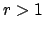and, then the mode is unique, and it is at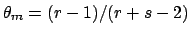. Depending on the value of the parameters the Beta pdf can take a large variety of shapes. For example, for large values of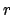and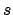, the function is very similar to a Gaussian distribution, while a constant function is obtained for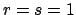. Using the Beta pdf as prior function in inferential problems with a binomial likelihood, we have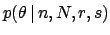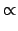(98)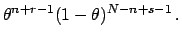(99)

The posterior distribution is still a Beta withand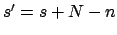, and expectation and standard deviation can be calculated easily from Eqs. (96) and (97). These formulae demonstrate how the posterior estimates become progressively independent of the prior information in the limit of large numbers; this happens when both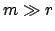and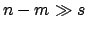. In this limit, we get the same result as for a uniform prior ().

 likelihood conjugate prior posterior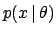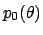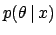Normal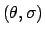Normal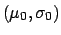Normal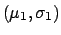[Eqs. (30)-(32)] Binomial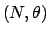Beta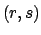BetaPoisson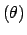GammaGamma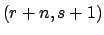Multinomial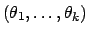Dirichlet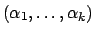Dirichlet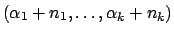Table 2 lists some of the more useful conjugate priors. For a more complete collection of conjugate priors, see e.g. (Bernardo and Smith 1994, Gelman et al 1995).Next: General principle based priors Up: Choice of priors - Previous: Purely subjective assessment of
Giulio D'Agostini 2003-05-13